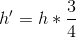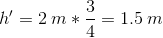## Example Questions

### Example Question #1 : How To Find The Length Of An Edge Of A Prism

The length of a box is 3 times the width. Which of the following gives the length (L inches) in terms of the width (W inches) of the box?

L = 3/W

L = W + 3

L = ½ (3W)

L = 3W

L = 3W

Explanation:

When reading word problems, there are certain clues that help interpret what is going on. The word “is” generally means “=” and the word “times” means it will be multiplied by something. Therefore, “the length of a box is 3 times the width” gives you the answer: L = 3 x W, or L = 3W.

### Example Question #1 : How To Find The Length Of An Edge Of A Prism

The width of a box, in inches, is 5 inches less than three times its length. Which of the following equations gives the width, W inches, in terms of the length, L inches, of the box?

W=5L-3

W=3-5L

W=5-3L

W=3L-5

W=3L-5

Explanation:

We notice the width is “5 inches less than three times its width,” so we express W as being three times its width (3L) and 5 inches less than that is 3L minus 5. In this case, W is the dependent and L is the independent variable.

W = 3L - 5

### Example Question #3 : Prisms

Sturgis is in charge of designing a new exhibit in the shape of a rectangular prism for a local aquarium. The exhibit will hold alligator snapping turtles and needs to have a volume of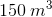. Sturgis knows that the exhibit will be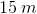long and go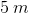back into the wall.

What will the height of the new exhibit be?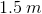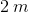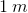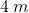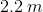Explanation:

This sounds like a geometry problem, so start by drawing a picture so that you know exactly what you are dealing with.

Because we are dealing with rectangular prisms and volume, we will need the following formula: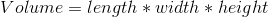OrWe are solving for height, so you can begin by rearranging the equation to getby itself: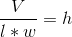Then, plug in our knowns (,and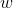)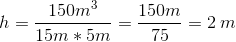Here is the problem worked out with a corresponding picture: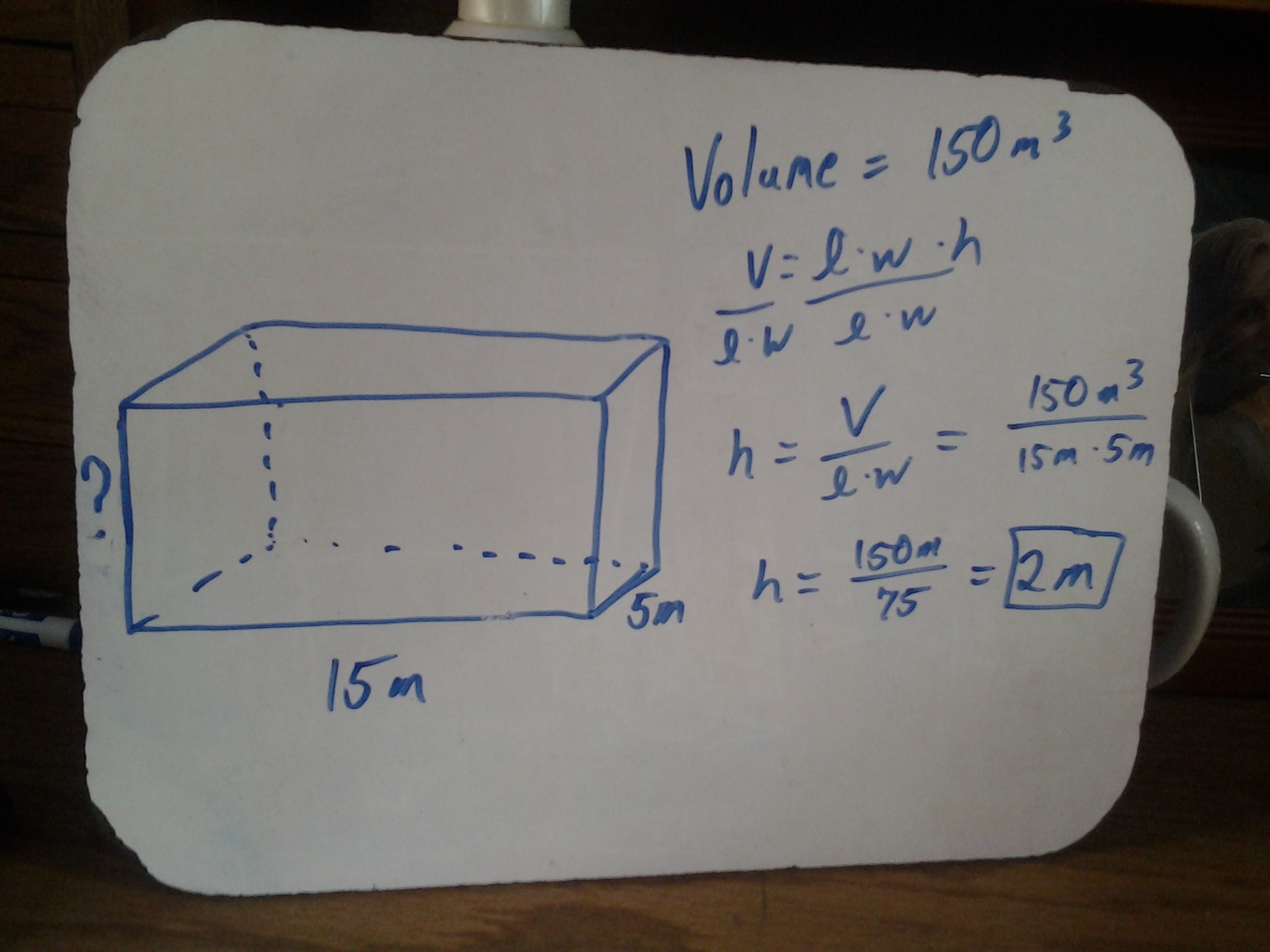### Example Question #4 : Prisms

Sturgis is in charge of designing a new exhibit in the shape of a rectangular prism for a local aquarium. The exhibit will hold alligator snapping turtles and needs to have a volume of. Sturgis knows that the exhibit will belong and goback into the wall.

If three-quarters of the exhibit's volume will be water, how high up the wall will the water come?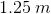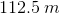Cannot be determined with the information provided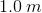Explanation:

The trickiest part of this question is the wording. This problem is asking for the height of the water in the exhibit if the exhibit is three-quarters full. We can find this at least two different ways.

1) The longer way requires that we begin by finding three quarters of the total volume: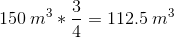Now we go back to our volume equation, and since we are again looking for height, we want it solved for: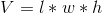Becomes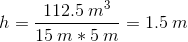2) The easier way requires that we recognize a key detail. If we take three-quarters of the volume without changing our length or width, our new height will just be three-quarters of the total height. We can solve for the total height of the exhibit by using the volume equation and rearranging it to solve for:At this point, we can substitute in our given values and solve for: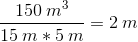So, the total height of the exhibit is. We can now easily solve for three-quarters of the total height: